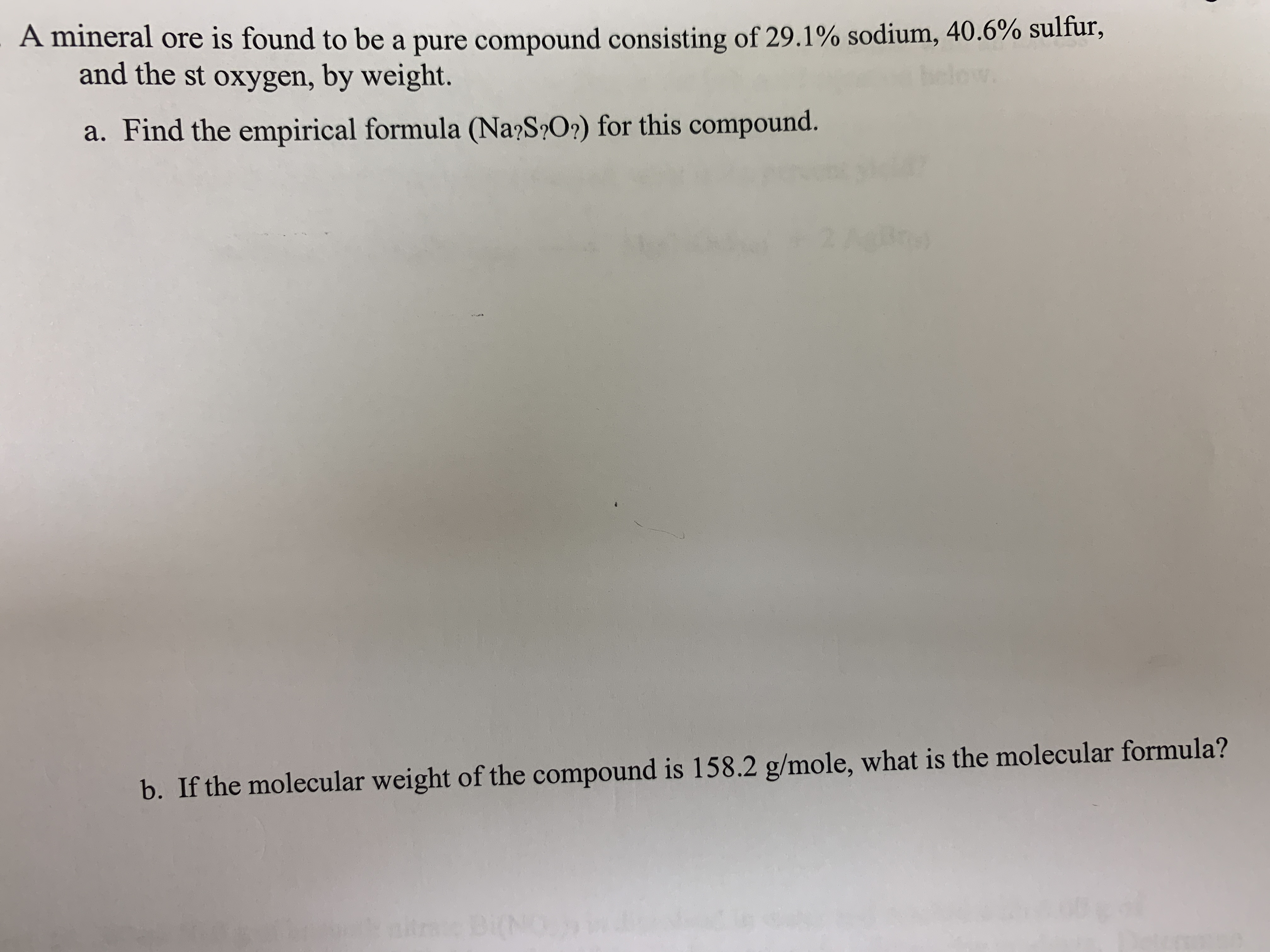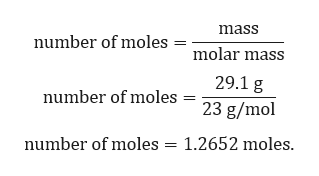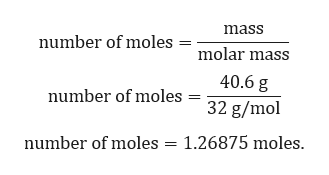# A mineral ore is found to be a pure compound consisting of 29.1% sodium, 40.6% sulfur,and the st oxygen, by weight.a. Find the empirical formula (Na?S O?) for this compound2 1b. If the molecular weight of the compound is 158.2 g/mole, what is the molecular formula?BiN

Question
1 viewshelp_outlineImage TranscriptioncloseA mineral ore is found to be a pure compound consisting of 29.1% sodium, 40.6% sulfur, and the st oxygen, by weight. a. Find the empirical formula (Na?S O?) for this compound 2 1 b. If the molecular weight of the compound is 158.2 g/mole, what is the molecular formula? BiN fullscreen
check_circle

Step 1

Given:

Percent mass of sodium = 29.1 %.

Percent mass of sulfur = 40.6 %.

Percent mass of oxygen = 100% - 29.1% -40.6 %= 30.3 %.

Conversion of mass percent to gram:

Since we are having mass percent, it is convenient to use 100 g of the compound as the starting material. Thus, in the 100 g of sample of above compound 29.1 g of sodium, 40.6 g of sulfur and 30.3 g of oxygen.

Step 2

Calculation for number moles of sodium:help_outlineImage Transcriptionclosemass number of moles molar mass 29.1 g number of moles 23 g/mol 1.2652 moles. number of moles fullscreen
Step 3

Calculation for number ...help_outlineImage Transcriptionclosemass number of moles molar mass 40.6 g number of moles 32 g/mol number of moles 1.26875 moles. fullscreen

### Want to see the full answer?

See Solution

#### Want to see this answer and more?

Solutions are written by subject experts who are available 24/7. Questions are typically answered within 1 hour.*

See Solution
*Response times may vary by subject and question.
Tagged in

### General Chemistry